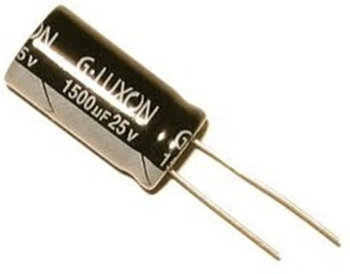﻿ What does the Voltage Rating on a Capacitor Mean?# What does the Voltage Rating on a Capacitor Mean?The voltage rating on a capacitor is the maximum amount of voltage that a capacitor can safely be exposed to and can store.

Remember that capacitors are storage devices. The main thing you need to know about capacitors is that they store X charge at X voltage; meaning, they hold a certain size charge (1µF, 100µF, 1000µF, etc.) at a certain voltage (10V, 25V, 50V, etc.). So when choosing a capacitor you just need to know what size charge you want and at which voltage.

Why does a capacitor come in different voltage ratings? Because you may need different voltages for a circuit depending on what circuit you're dealing with. Remember, capacitors supply voltage to a circuit just like a battery does. The only difference is a capacitor discharges its voltage much quicker than a battery, but it's the same concept in how they both supply voltage to a circuit. A circuit designer wouldn't just use any voltage for a circuit but a specific voltage which is needed for the circuit. For one circuit, 12 volts may be needed. A capacitor with a 12V rating or higher would be used in this case. In another, 50 volts may be needed. A capacitor with a 50V rating or higher would be used. This is why capacitors come in different voltage ratings, so that they can supply circuits with different voltages, fitting the power (voltage) needs of the circuit.

Take note that a capacitor's voltage rating is not the voltage that the capacitor will charge up to, but only the maximum amount of voltage that a capacitor should be exposed to and can store safely. For the capacitor to charge up to the desired voltage, the circuit designer must design the circuit specificially for the capacitor to charge up to that voltage. A capacitor may have a 50-volt rating but it will not charge up to 50 volts unless it is fed 50 volts from a DC power source. The voltage rating is only the maximum voltage that a capacitor should be exposed to, not the voltage that the capacitor will charge up to. A capacitor will only charge to a specific voltage level if fed that level of voltage from a DC power source.

Keep in mind that a good rule for choosing the voltage ratings for capacitors is not to choose the exact voltage rating that the power supply will supply it. It is normally recommended to give a good amount of room when choosing the voltage rating of a capacitor. Meaning, if you want a capacitor to hold 25 volts, don't choose exactly a 25 volt-rated capacitor. Leave some room for a safety margin just in case the power supply voltage ever increased due to any reasons. If you measured the voltage of a 9V battery supply, you would notice that it reads above 9 volts when it's new and has full life. If you used an exact 9-volt rated capacitor, it would be exposed to a higher voltage than the maximum specified voltage (the voltage rating). Usually, in a case such as this, it shouldn't be a problem, but nevertheless, it's a good safety margin and engineering practice to do this. You can't really go wrong choosing a higher voltage-rated capacitor than the voltage that the power supply will supply it, but you can definitely go wrong choosing a lower voltage-rated capacitor than the voltage that it will be exposed to. If you charge up a capacitor with a lower voltage rating than the voltage that the power supply will supply it, you risk the chance of the capacitor exploding and becoming defective and unusable. So don't expose a capacitor to a higher voltage than its voltage rating. The voltage rating is the maximum voltage that a capacitor is meant to be exposed to and can store. Some say a good engineering practice is to choose a capacitor that has double the voltage rating than the power supply voltage you will use to charge it. So if a capacitor is going to be exposed to 25 volts, to be on the safe side, it's best to use a 50 volt-rated capacitor.

Also, note that the voltage rating of a capacitor is also referred to at times as the working voltage or maximum working voltage (of the capacitor). So when seeing the (maximum) working voltage specification on a datasheet, this value refers to the maximum continouous voltage that a capacitor can withstand without becoming damaged.